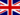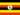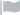### This Course has been revised!

For a more enjoyable learning experience, we recommend that you study the mobile-friendly republished version of this course.

Take me to revised course.

- or -

Continue studying this course

# How to calculate magnification

• Study Notes
• Discuss This TopicAngela A. 2 0 Each lens has a magnifcation factor. The Eye piece is x10 and the Objective lens is x40. To calculate an objects magnification is to multiply the Eye piece and the objective lens, this is equal to x400 magnification.Angela A. 0 0 why is this not in addition but in multiplication.Odongo M. 0 0 Biology -> How to calculate magnification How to calculate magnificationSusan G. 0 0 Each lens has a magnification factor. The object lens has x 40. The eyepiece on its own is x 10. This makes the magnification of thee microscope is 400.Hossam E. 0 0 niceDon M. 0 0 When calculating magnification is there a way to magnify without straining visual?
• Text Version